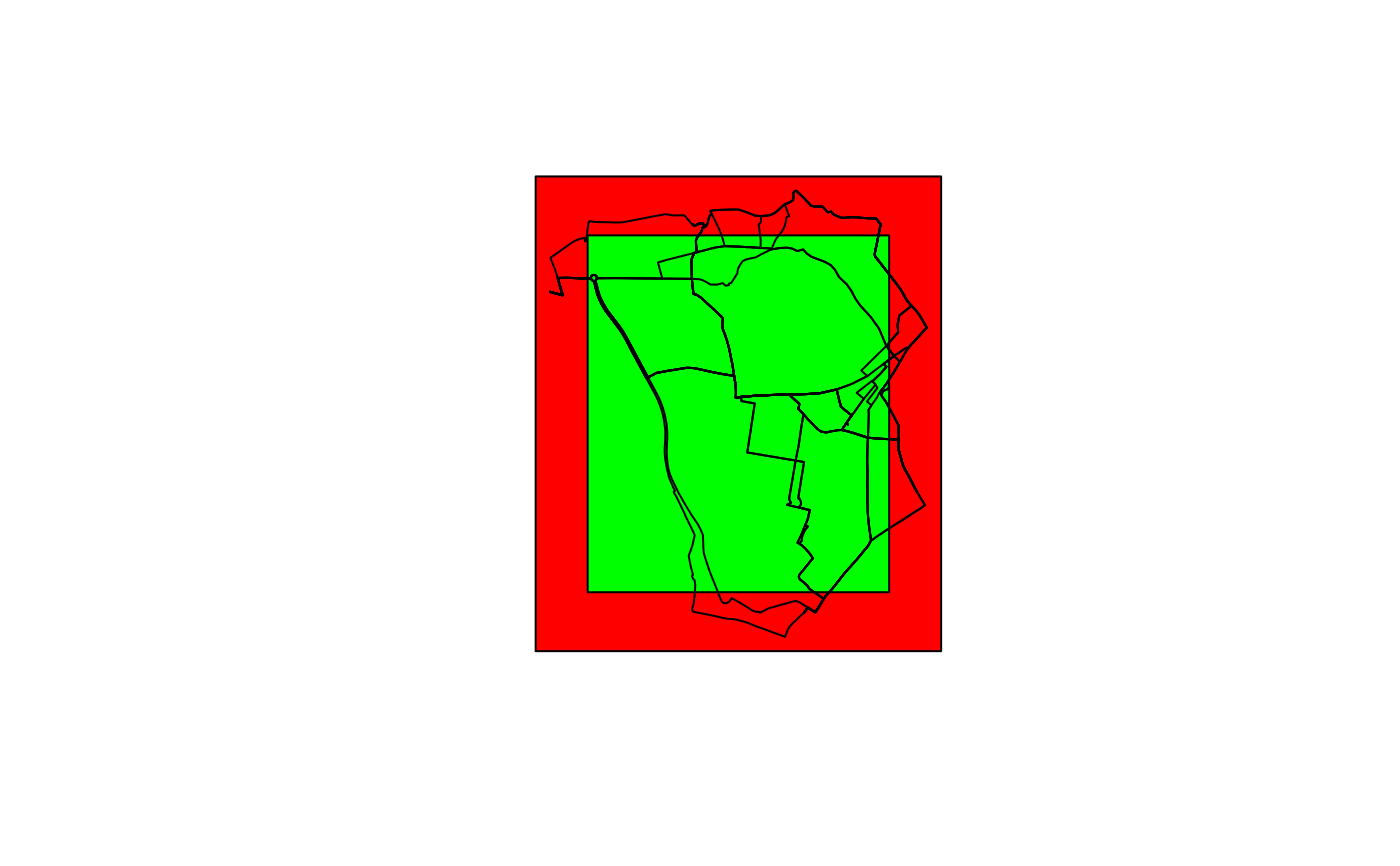Takes a geographic object or bounding box as an input and outputs a bounding box, represented as a bounding box, corner points or rectangular polygon.

## Usage

``````geo_bb(
shp,
scale_factor = 1,
distance = 0,
output = c("polygon", "points", "bb")
)``````

## Arguments

shp

Spatial object

scale_factor

Numeric vector determining how much the bounding box will grow or shrink. Two numbers refer to extending the bounding box in x and y dimensions, respectively. If the value is 1, the output size will be the same as the input.

distance

Distance in metres to extend the bounding box by

output

Type of object returned (polygon by default)

bb_scale

Other geo: `bbox_scale()`, `bind_sf()`, `geo_bb_matrix()`, `geo_buffer()`, `geo_length()`, `geo_projected()`, `geo_select_aeq()`, `quadrant()`

## Examples

``````shp <- routes_fast_sf
shp_bb <- geo_bb(shp, distance = 100)
plot(shp_bb, col = "red", reset = FALSE)
plot(geo_bb(routes_fast_sf, scale_factor = 0.8), col = "green", add = TRUE)